simulink
• 基于matlab实现的自适应中值滤波器，基于matlab实现的自适应中值滤波器
• matlab图像处理自适应中值滤波，function, 可运行，很好很好很好
• 图像处理作业，自适应中值滤波器的实现，根据数字图像处理 第三版（冈萨雷斯 著）此书所描述的算法实现编码
• RT,看到有matlab实现，参照写了C++实现，vs环境需要配置opencv
• 自适应中值滤波算法 参考文献：Chan R H, Ho C W, Nikolova M. Salt-and-pepper noise removal by median-type noise detectors and detail-preserving regularization[J]. IEEE Trans. Image Process, 2005, 14(10)...椒盐去噪
• 用于图像去除噪声的自适应中值滤波算法,能够有效的去除图像中的噪声达到图像复原的效果，对于学习图像复原和图像处理有很大的帮助
• 自己实现的自适应中值滤波，代码绝对没有问题，欢迎指正
• 结果对比二、自适应中值滤波1. 原理部分2.程序代码3. 结果对比 一、自适应均值滤波器 1. 原理部分： 加入噪声： 原理： 将图片灰度化，然后将图片像素归一化到[0，1]之间，然后添加均值为0，方差为0.1的高斯噪声，...


matlab编程实现自适应滤波器
一、自适应均值滤波器1. 原理部分：2. 程序代码3. 结果对比
二、自适应中值滤波1. 原理部分2.程序代码3. 结果对比

一、自适应均值滤波器
1. 原理部分：
加入噪声： 原理： 将图片灰度化，然后将图片像素归一化到[0，1]之间，然后添加均值为0，方差为0.1的高斯噪声，乘以255映射回图片原来的灰度级，用max(0,min(t1,255))操作将超出[0，255]的纠正。效果如图：随机变量最简单的统计量是均值和方差，这些适当的参数是自适应局部滤波器的基础。均值给出了计算均值的区域中灰度平均值的度量,而方差给出了这个区域的平均对比度的度量。2. 程序代码
主函数：
clear;clc;
%% 导入原图像，并添加高斯噪声
image=rgb2gray(image);	%灰度化
[m,n,~]=size(image);
% y=0+0.2*randn(m,n/2);	%只添加一半
y=0+0.2*randn(m,n);		%全范围加上噪声
%先将其double化，再除以255 便于后面计算
t1=double(image)/255;
%加上噪声
% t1(:,(n/2+1):n)=t1(:,(n/2+1):n)+y;
t1(:,:)=t1(:,:)+y;
t1 = max(0,min(t1,1));  %处理到[0,1]
% t1(:,:)=t1(:,:)+y2;
%将像素范围扩大至0--255
t1=t1*255;
%转换为uint8类型  包含噪声的图片
t2=uint8(t1);
figure("color",[1,1,1])
subplot(1,2,1),imshow(image),title('原图');
subplot(1,2,2),imshow(t2),title('加入均值为0，标准差为0.2的高斯噪声后');

%% 计算噪声方差
t2_reshape=reshape(t1,1,1,length(t1(:)));
global_means=mean(t2_reshape);
global_vars=var(t2_reshape,1);

%% 自适应均值滤波器
image_with_noise=t1;
image1=double(image);
%扩展周围一圈，因为滤波器为7x7，可以有镜像扩充、对称扩充、常数扩充等等

imagemean=image_expand; %存储每个位置的局部均值
imagevar=image_expand;  %存储每个位置的局部方差

[width,height,z]=size(image_expand);
s=7;    %滤波器阶数
for i=1:width-s+1
for j=1:height-s+1
box=image_expand(i:i-1+s,j:j-1+s);
boxs=reshape(box,1,1,length(box(:)));
means=mean(boxs);   %求均值
imagemean(i+(s-1)/2,j+(s-1)/2)=means;
vars=var(boxs,1);   %求方差
imagevar(i+(s-1)/2,j+(s-1)/2)=vars;
end
end
image_expand=image_expand(3+1:width-3,3+1:height-3);
imagemean=imagemean(3+1:width-3,3+1:height-3);	%去掉扩充的边缘
imagevar=imagevar(3+1:width-3,3+1:height-3);	%去掉扩充的边缘
noise = mean2(imagevar);%估计全局噪声方差

%% 书上公式计算得到的滤波结果
image_new=image_expand-(noise./imagevar.*(image_expand-imagemean));
image_new = max(0,min(image_new,255));  %处理到[0,255]
image_new=uint8(image_new);

%% matlab源码做了改进，效果差距不是很大
image_new_default=imagemean + (max(0, imagevar - noise) ./max(imagevar, noise)) .* (image_expand - imagemean);
image_new_default=uint8(image_new_default);
%% 画图
figure("color",[1,1,1])
subplot(2,2,1);
imshow(image);
imshow(t2);
title('原图像');
output=mean_filter(image_expand,7);
subplot(2,2,2);
imshow(output);
title('均值滤波处理后结果');

subplot(2,2,3);
imshow(image_new);
title('自适应均值滤波处理后结果');

subplot(2,2,4);
imshow(image_new_default);
title('修改后的自适应均值滤波处理后结果');

% figure("color",[1,1,1])
% image_global_var=image_expand-(global_vars./imagevar.*(image_expand-imagemean));
% image_global_var = max(0,min(image_global_var,255));  %处理到[0,255]
% image_global_var=uint8(image_global_var);
% imshow(image_global_var);
% title('global var自适应均值滤波处理后结果');


子函数：
%自编写均值滤波器
function output=mean_filter(image,n)
%输入image为原始图像，n为滤波器规模n*n，输出为滤波后的图像output
[h,w]=size(image);
imaged=double(image); %转换为double类型
imagedd=imaged;  %初始化滤波结果的规模
a=ones(n,n);
for i=1:h-n+1
for j=1:w-n+1
pattern=imaged(i:i+n-1,j:j+n-1).*a;
means=sum(sum(pattern));
imagedd(i+(n-1)/2,j+(n-1)/2)=means/(n*n);
end
end
output=uint8(imagedd);
end


3. 结果对比二、自适应中值滤波
1. 原理部分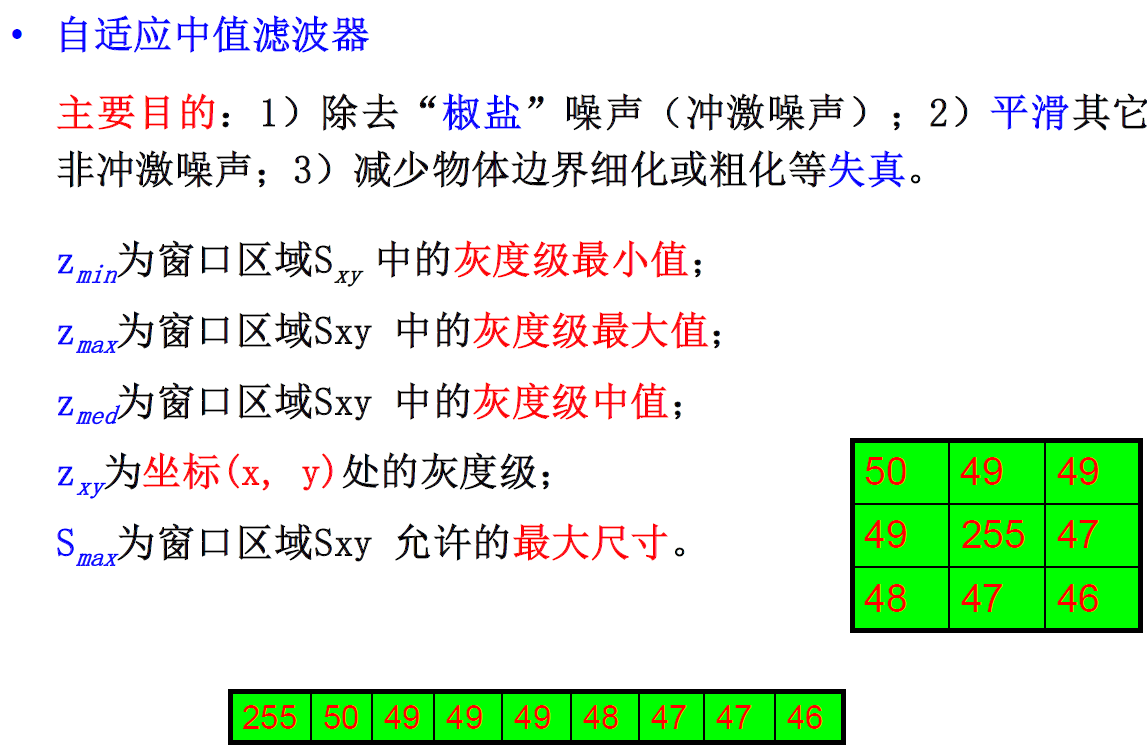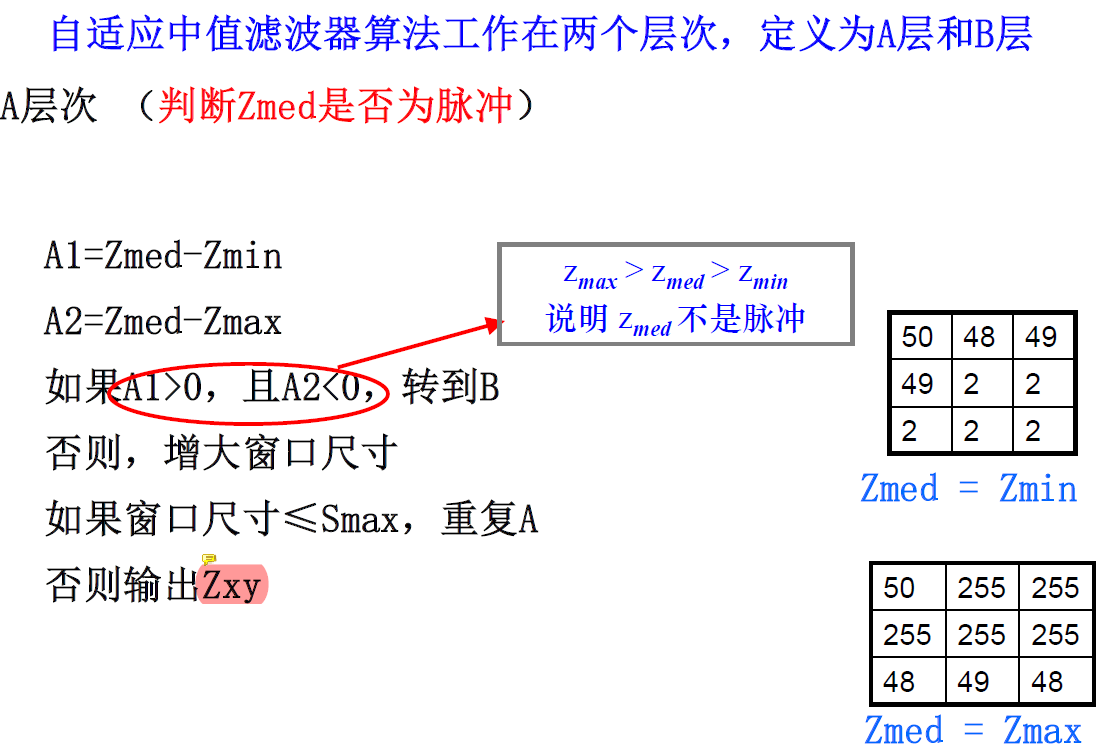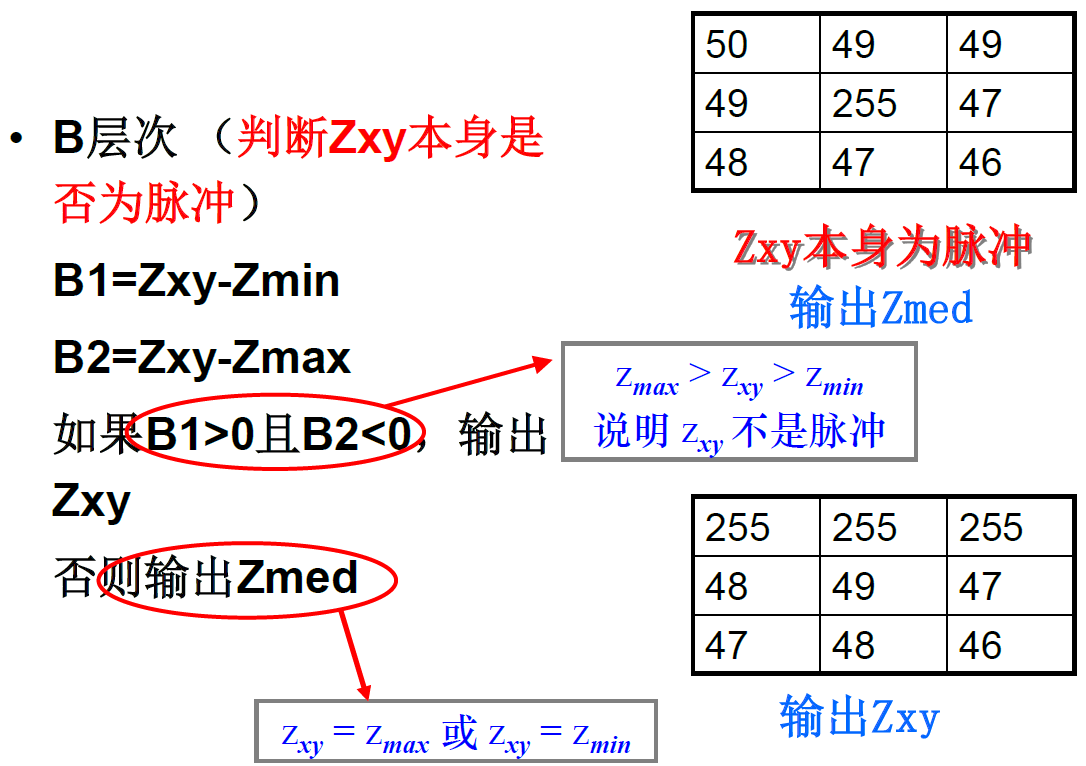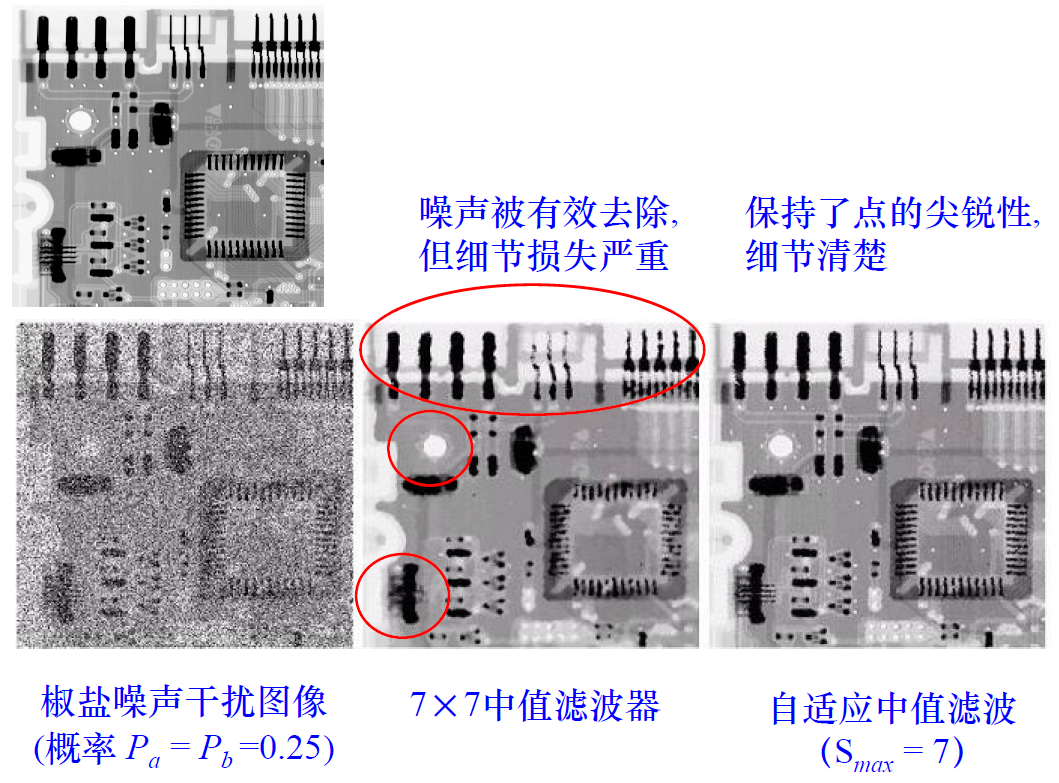2.程序代码
主程序：
gray=imread('5.png');
gray=rgb2gray(gray);	%灰度化
%% 加入噪声
gray_noise=imnoise(gray,'salt & pepper',0.5);
%% 中值滤波
M1 = medfilt2(gray_noise,[3,3]);
%% 自适应中值滤波
%%
figure("color",[1,1,1]);
subplot(131)
imshow(gray_noise)
title("gray with noise")
subplot(132)
imshow(M1)
title("中值滤波")
subplot(133)
imshow(f1)
title("自适应中值滤波")

子函数：
function f = adaptive_median_filter (g, Smax)
%% 判断邻域是否合理
if (Smax <= 1) || (Smax/2 == round(Smax/2)) || (Smax ~= round(Smax))
error ('SMAX must be an odd integer > 1.')
end
%%
f = g;
f(:) = 0;
%% 标记是否已处理过
%% 开始自适应滤波
for k = 3:2:Smax
zmin = ordfilt2(g, 1, ones(k, k),'symmetric');
zmax = ordfilt2(g, k * k, ones(k, k), 'symmetric');
zmed = medfilt2(g, [k k], 'symmetric');
%% 判断是否进入进程B
processUsingLevelB  = (zmed > zmin) & (zmax > zmed) & ~alreadyProcessed;
%% 若g不是脉冲，保留原值
zB = (g > zmin) & (zmax > g);
outputZxy = processUsingLevelB & zB;
%% 若是脉冲，用Zmed替换
outputZmed = processUsingLevelB & ~zB;
f (outputZxy) = g(outputZxy);
f (outputZmed) = zmed(outputZmed);
%% 已处理记录
%% 是否退出
break;
end
end
%% 大于窗口尺寸后，Zxy替换成Zmed输出
end

3. 结果对比
噪声密度0.2：噪声密度0.5：由图可知，当脉冲噪声的空间密度过大时，中值滤波的性能就不是很好，自适应中值滤波可以处理具有更大概率的脉冲噪声。自适应中值滤波平滑非脉冲噪声时试图保留细节，这是 中值滤波器做不到的。
展开全文• 开始之前 在上一篇我们实现了读取噪声图像, 然后 进行三种形式的均值滤波得到结果, 由于我们自己写的均值滤波未作边缘处理, 所以效果有一定的下降,...首先介绍了中值滤波的原理, 给出其实现思路,并根据思路实现了 C+...
开始之前
在上一篇我们实现了读取噪声图像, 然后 进行三种形式的均值滤波得到结果, 由于我们自己写的均值滤波未作边缘处理, 所以效果有一定的下降, 但是总体来说, 我们得到的结果能够说明我们的算法执行之后得到的图像噪声更低, 图像更清晰. 但是也会造成图像的模糊, 导致部分细节丢失. 在这一章中,我们介绍一下中值滤波及其实现
摘要
首先介绍了中值滤波的原理, 给出其实现思路,并根据思路实现了 C++ 的代码, 然后 同样测试 opencv 自带的中值滤波, 同样的测试图像, 得到对比结果, 分析代码的实现过程, .
正文
中值滤波原理
中值滤波(Media Filter)就是对于图像的每一个点计算其邻域窗口的像素序列中值, 可以表示为:

g

(

x

,

y

)

=

m

e

i

d

a

(

i

,

j

)

∈

S

f

(

i

,

j

)

g(x,y) = meida_{(i,j) \in S}f(i,j)

核心就是将相应窗口内的像素值进行排列, 我们之前也说过, 我们选择的窗口为奇数尺寸, 所以我们能够保证窗口内的像素个数也是奇数个, 这样我们可以保证取得唯一的中值, 相应的设置为该点的目标值就行了.
C++ 实现中值滤波
我们来实现一下, 这方面还是能够找到不少结果的, 感觉这个博主写的还是很不错的,有兴趣的可以看下数字图像处理------中值滤波,还有图像处理之中值滤波介绍及C实现, 或者 中值滤波器（Median filter）特性及其实现, 这里我就不再造轮子了, 我们来看下 C++的实现 , 主要参考 第一篇文章, 可以看下效果
这里有一点点需要讨论的, 对于彩色图像的三个通道怎么处理, 自己的思路就是分成三个通道进行处理, 然后分别得到三个图之后进行合并三个通道, 得到结果图像. 查了下 目测大家都是这么做的, 可以看OpenCV 彩色图像的自适应中值滤波 C++ 和 彩色图像空间滤波（MATLAB） 这两篇文章, 思路都是一样的, 我们来实现一下.
//中值滤波：C++ 代码实现 // 处理单通道图像 // 参考 https://www.cnblogs.com/ranjiewen/p/5699395.html
cv::Mat medianFilterGray(const cv::Mat &src, int ksize = 3)
{
cv::Mat dst = src.clone();
//0. 准备：获取图片的宽，高和像素信息，
const int  num = ksize * ksize;
std::vector<uchar> pixel(num);

//相对于中心点，3*3领域中的点需要偏移的位置
int delta[3 * 3] = {
{ -1, -1 }, { -1, 0 }, { -1, 1 }, { 0, -1 }, { 0, 0 }, { 0, 1 }, { 1, -1 }, { 1, 0 }, {1, 1}
};
//1. 中值滤波，没有考虑边缘
for (int i = 1; i < src.rows - 1; ++i)
{
for (int j = 1; j < src.cols - 1; ++j)
{
//1.1 提取领域值 // 使用数组 这样处理 8邻域值 不适合更大窗口
for (int k = 0; k < num; ++k)
{
pixel[k] = src.at<uchar>(i+delta[k], j+ delta[k]);
}
//1.2 排序  // 使用自带的库及排序即可
std::sort(pixel.begin(), pixel.end());
//1.3 获取该中心点的值
dst.at<uchar>(i, j) = pixel[num / 2];
}
}
return dst;
}

思路还是那个思路, 不过在写的过程中, 我在想, 能不能直接处理彩色的图像呢, 对于彩色图像最麻烦的地方就是排序了, 我们没办法考虑颜色的高低值, 所以 那我们自定义一个比较函数应该就行了吧. 我们使用三个颜色的和值 做比较 这里使用了C++ 的sort 自定义函数的方法, 这边采用的比较函数的方式, 还有别的方式实现两个元素的比较, 可以参考c++中vector自定义排序的问题
// 自定义两个像素的比较函数,  // 使用和值 排序
bool comp(const cv::Vec3b &p1, const cv::Vec3b &p2)
{
return (p1 + p1 + p1) < (p2 + p2 + p2);
}
// 尝试彩色图像, 中值排序使用三个通道的和排序
cv::Mat medianFilterColor(const cv::Mat &src, int ksize = 3)
{
cv::Mat dst = src.clone();
//0. 准备：获取图片的宽，高和像素信息，
const int  num = ksize * ksize;
std::vector<cv::Vec3b> pixel(num);

//相对于中心点，3*3领域中的点需要偏移的位置
int delta[3 * 3] = {
{ -1, -1 }, { -1, 0 }, { -1, 1 }, { 0, -1 }, { 0, 0 }, { 0, 1 }, { 1, -1 }, { 1, 0 }, {1, 1}
};
//1. 中值滤波，没有考虑边缘
for (int i = 1; i < src.rows - 1; ++i)
{
for (int j = 1; j < src.cols - 1; ++j)
{
//1.1 提取领域值 // 使用数组 这样处理 8邻域值 不适合更大窗口
for (int k = 0; k < num; ++k)
{
pixel[k] = src.at<cv::Vec3b>(i + delta[k], j + delta[k]);
}
//1.2 排序  // 使用自定义的排序函数排序彩色图像
std::sort(pixel.begin(),pixel.end(),comp);
//1.3 获取该中心点的值
dst.at<cv::Vec3b>(i, j) = pixel[num / 2];
}
}
return dst;
}


opencv 中值滤波
这里还是之前的方法, 一样的接口, 实现起来很简单, opencv 提供的 函数还是很丰富的, 很厉害
// opencv 中值滤波
cv::Mat mediaFilterDefault(const cv::Mat &src, int ksize = 3)
{
cv::Mat dst;
cv::medianBlur(src, dst, ksize);
return dst;
}

中值滤波算法对比
我们这里就跟之前均值算法的计算很相似了, 我们已经写了三种算法的实现, 然后测试就好了, 趁着功夫, 将上一章一直重复的两个图比较并输出参数的部分写成了一个函数
// 对比两个图像 然后输出 参数信息
QString compareImages(const cv::Mat &I1,
const cv::Mat &I2,
const QString str = "noise",
const QString str_temp = "image-%1: psnr:%2, mssim: B:%3 G:%4 R:%5")
{
double psnr_ = getPSNR(I1, I2);
cv::Scalar mssim_ = getMSSIM(I1, I2);

// 根据 输出模板 生成参数信息
QString res_str = str_temp.arg(str)
.arg(psnr_)
.arg(mssim_.val)
.arg(mssim_.val)
.arg(mssim_.val);

return res_str;
// cv::imwrite(IMAGE_DIR + "dst_" + std::to_string(i + 1) + ".png", dst[i]);
}

没什么难度, 就是用来拼接一个字符串, 用来显示在界面上, 或者 输出输出来,
这样的我们就能很容易的去写测试的函数了, 三种方法依次去实现, 比较麻烦的是第一种, 需要将彩色图像分成三个通道的灰度图像, 然后分别进行中值滤波, 最后合并结果,得到结果图像.
void MainWindow::testFunc2(void)
{
// 测试 中值 滤波 三种方式的不同
const int TEST = 1; // 使用统一的图进行测试 暂时使用 高 椒盐噪声图像
QString res_str;

// 噪声图像的参数值
res_str = compareImages(gSrcImg, gNoiseImg[TEST]);
ui->pt_log->appendPlainText(res_str);

cv::Mat test_img = gNoiseImg[TEST];

cv::Mat dst;

// 测试 中值滤波 拆分三个通道进行中值滤波然后合并图像
std::vector<cv::Mat> bgr(3);
cv::split(test_img, bgr);
bgr = medianFilterGray(bgr);
bgr = medianFilterGray(bgr);
bgr = medianFilterGray(bgr);

cv::merge(bgr, dst);     // 第一种方式
dst = medianFilterColor(test_img);   // 第二种 彩色直接 计算中值滤波
dst = mediaFilterDefault(test_img);  // opencv 实现 中值滤波

// 分别计算三种方式得到的滤波的效果 (结果图与 原始图比较)
for(int i=0;i<3;i++)
{
res_str = compareImages(gSrcImg, dst[i]);
// 噪声的参数值
ui->pt_log->appendPlainText(res_str);

cv::imwrite(IMAGE_DIR + "dst_media_" + std::to_string(i+1)+".png",dst[i]);
}
}

我们仍然选择高椒盐噪声图像用于测试, 先看下结果, 分别对应噪声图的参数, 以及三种方法进行的参数结果. 第三行的结果就是我们进行自定义排序的图像处理,
image-noise: psnr:19.4727, mssim: B:0.353134 G:0.383638 R:0.629353
image-noise: psnr:33.3725, mssim: B:0.896859 G:0.915976 R:0.912563
image-noise: psnr:31.2668, mssim: B:0.866162 G:0.901717 R:0.879337
image-noise: psnr:34.3125, mssim: B:0.902338 G:0.921419 R:0.91531

我们看一下结果图像, 原始图像可以看 https://gitee.com/schen00/BlogImage/raw/master/image/1588468343599.png 这里,

gitee 限制了 1M 以上的图的显示, 所以有需要的去看这个就好.

最近一直用的图拼接使用的 做好图 在线拼接图片 主要是懒得自己写了, http://www.zuohaotu.com/image-merge.aspx 链接在这里了 有需要自取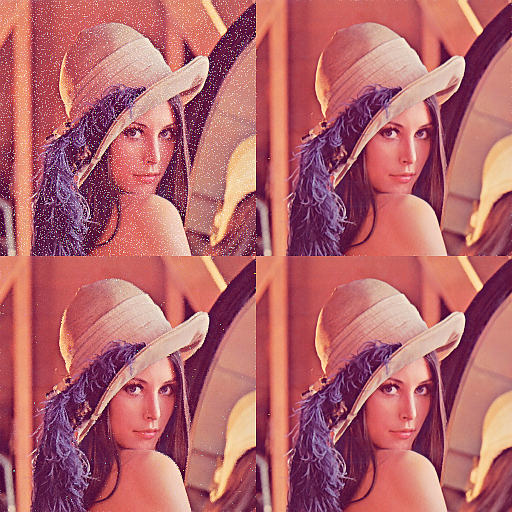这里的第一副图是噪声图像, 第二副是我们拆分通道处理后拼接起来了的, 没有处理边缘的细节问题, 第三章图就是我们进行自定义中值排序得到的图, 部分点处理不掉 甚至还复制了出来, 不过整体效果还是不错的, 第四章图就是opencv 自带的中值滤波的处理.
中值滤波算法优化
类似均值滤波, 处理的时候考虑变化了的边界就好了, 那中值滤波怎么优化呢, 感觉这一块做的人还挺多, 中值滤波的优化主要是使用自适应中值滤波, 和在中值滤波的方法上进行加速运算,
自适应中值滤波
可以参考自适应中值滤波及实现, 我感觉介绍的还是比较详细的, 主要的思路就是如果噪声比较严重时, 窗口获取到的中值可能是噪声值, 这时候增大窗口, 然后重新进行中值滤波,直到找到比较符合的中值. 引用他给出的部分叙述

在自适应中值滤波算法中，A步骤里面会先判断是否满足

Z

m

i

n

<

Z

m

e

d

<

Z

m

a

x

Z

m

i

n

<

Z

m

e

d

<

Z

m

a

x

Zmin<Zmed<ZmaxZmin<Zmed<Zmax

。这一步骤实质是判断当前区域的中值点是否是噪声点，通常来说是满足

Z

m

i

n

<

Z

m

e

d

<

Z

m

a

x

Z

m

i

n

<

Z

m

e

d

<

Z

m

a

x

Zmin<Zmed<ZmaxZmin<Zmed<Zmax

这个条件的，此时中值点不是噪声点，跳转到B；考虑一些特殊情况，如果

Z

m

e

d

=

Z

m

i

n

Z

m

e

d

=

Z

m

i

n

或

者

Z

m

e

d

=

Z

m

a

x

Z

m

e

d

=

Z

m

a

x

Zmed=ZminZmed=Zmin或者Zmed=ZmaxZmed=Zmax

，则认为是噪声点，应该扩大窗口尺寸，在一个更大的范围内寻找一个合适的非噪声点，随后再跳转到B，否则输出的中值点是噪声点； 接下来考虑跳转到B之后的情况：判断中心点的像素值是否是噪声点，判断条件为

Z

m

i

n

<

Z

x

y

<

Z

m

a

x

Z

m

i

n

<

Z

x

y

<

Z

m

a

x

Zmin<Zxy<ZmaxZmin<Zxy<Zmax

，原理同上，因为如果

Z

x

y

=

Z

m

i

n

Z

x

y

=

Z

m

i

n

Zxy=ZminZxy=Zmin

或者

Z

x

y

=

Z

m

a

x

Z

x

y

=

Z

m

a

x

Zxy=ZmaxZxy=Zmax

，则认为是噪声点。如果不是噪声点，我们可以保留当前像素点的灰度值；如果是噪声点，则使用中值替代原始灰度值，滤去噪声。

同样的, 图像处理基础(2)：自适应中值滤波器(基于OpenCV实现), 这篇文章写的更好一点, 并给出了 opencv 的实现代码, 我们来看一下
// 自适应中值滤波窗口实现  // 图像 计算座标, 窗口尺寸和 最大尺寸
uchar adaptiveProcess(const Mat &im, int row, int col, int kernelSize, int maxSize)
{
std::vector<uchar> pixels;
for (int a = -kernelSize / 2; a <= kernelSize / 2; a++)
for (int b = -kernelSize / 2; b <= kernelSize / 2; b++)
{
pixels.push_back(im.at<uchar>(row + a, col + b));
}
sort(pixels.begin(), pixels.end());
auto min = pixels;
auto max = pixels[kernelSize * kernelSize - 1];
auto med = pixels[kernelSize * kernelSize / 2];
auto zxy = im.at<uchar>(row, col);
if (med > min && med < max)
{
// to B
if (zxy > min && zxy < max)
return zxy;
else
return med;
}
else
{
kernelSize += 2;
if (kernelSize <= maxSize)
return adaptiveProcess(im, row, col, kernelSize, maxSize); // 增大窗口尺寸，继续A过程。
else
return med;
}
}
// 自适应均值滤波
cv::Mat adaptiveMediaFilter(const cv::Mat &src, int ksize = 3)
{
int minSize = 3; // 滤波器窗口的起始尺寸
int maxSize = 7; // 滤波器窗口的最大尺寸
cv::Mat dst;
// 扩展图像的边界
cv::copyMakeBorder(src, dst, maxSize / 2, maxSize / 2, maxSize / 2, maxSize / 2, cv::BorderTypes::BORDER_REFLECT);
// 图像循环
for (int j = maxSize / 2; j < dst.rows - maxSize / 2; j++)
{
for (int i = maxSize / 2; i < dst.cols * dst.channels() - maxSize / 2; i++)
{
dst.at<uchar>(j, i) = adaptiveProcess(dst, j, i, minSize, maxSize);
}
}
cv::Rect r = cv::Rect(cv::Point(maxSize / 2, maxSize / 2), cv::Point(dst.rows-maxSize / 2, dst.rows-maxSize / 2));
cv::Mat res = dst(r);
return res;
}

我们这里还是使用的分离三个通道然后进行自适应均值滤波, 参数就使用默认的3, 最大窗口设为7, 我们测试还是跑的之前的高椒盐噪声图像, 下面给出的最后一行就是我们使用自适应中值滤波得到的结果, 至少从 psnr 的参数上我们能看到图像质量的提升, 我们给出图像结果, 肉眼上能看出稍微一点的区别, 对比之前的已经完全不存在白点了, 图像已经比较接近真实图像了…
// 拆分三个通道 计算自适应中值滤波
cv::split(test_img, bgr);
for (int i = 0; i < 3; i++)
cv::merge(bgr, dst);

image-noise: psnr:19.4727, mssim: B:0.353134 G:0.383638 R:0.629353
image-noise: psnr:33.3725, mssim: B:0.896859 G:0.915976 R:0.912563
image-noise: psnr:31.2655, mssim: B:0.86636 G:0.901517 R:0.879384
image-noise: psnr:34.3125, mssim: B:0.902338 G:0.921419 R:0.91531
image-noise: psnr:37.4024, mssim: B:0.946158 G:0.958146 R:0.953884中值滤波计算加速
由于中值滤波无论多大的窗口都是用来将窗口内的像素进行排序, 这里的优化有两个方向 一个是窗口的优化, 一个计算的加速,
我真的 imageshop 的这篇文章 任意半径中值滤波（扩展至百分比滤波器）O(1)时间复杂度算法的原理、实现及效果。 已经写的比较完全了, 我都不想在写了,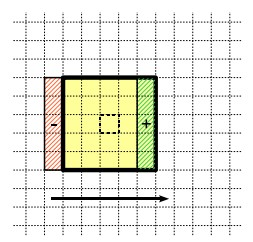再从中值滤波的快速算法 偷一张图,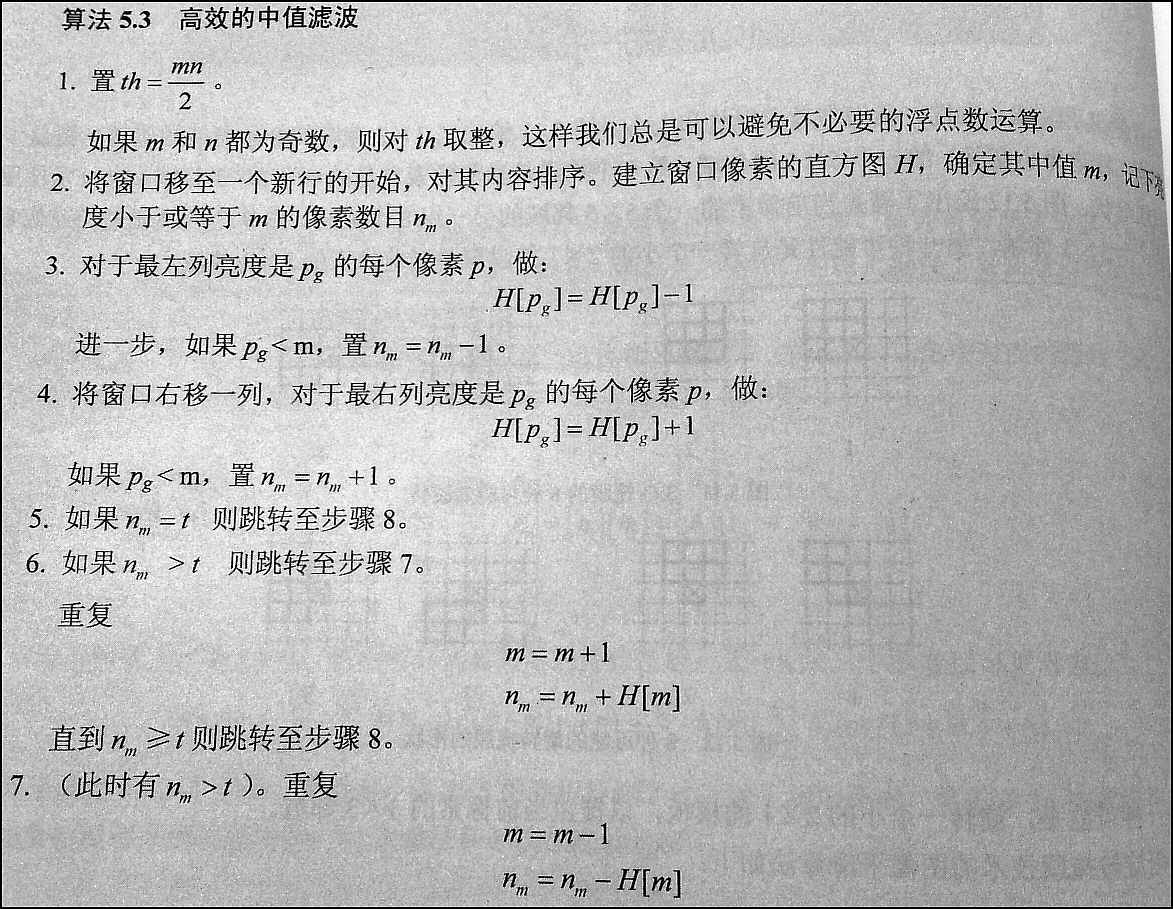感兴趣的可以看一下的链接 OpenCV源码分析（四）：中值滤波 这里详细介绍了 opencv 中怎么实现的 中值滤波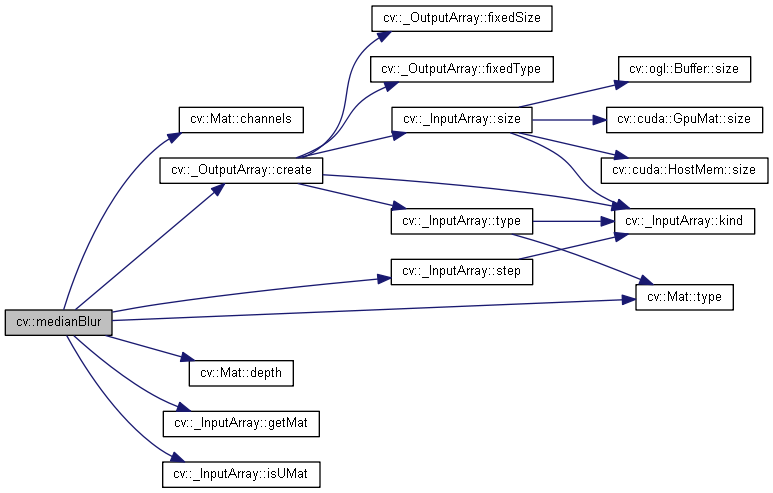总结
算是从中值滤波的基础上做了一个开始, 介绍了一下中值滤波的原理, 然后根据原理使用C++ 进行了实现, 之后再进行 opencv 的实现, 然后我们根据之前的程序上加入了中值滤波的实现效果, 最后在中值滤波的基础上进行优化, 做了自适应中值滤波的实现,测试发现结果还要更好, 最后我稍微提了一下中值滤波的优化加速, 这一块做的很多, 可以去参考里面去找, 算是完成了中值滤波的章节, 如果这里搞懂了我再来完善这一章节…
参考
《绘制函数调用图（call graph）（4）：doxygen + graphviz_运维_许振坪的专栏-CSDN博客》. 见于 2020年5月2日. https://blog.csdn.net/benkaoya/article/details/79763668.《任意半径中值滤波（扩展至百分比滤波器）O(1)时间复杂度算法的原理、实现及效果。 - Imageshop - 博客园》. 见于 2020年5月3日. https://www.cnblogs.com/Imageshop/archive/2013/04/26/3045672.html.《数字图像处理------中值滤波 - ranjiewen - 博客园》. 见于 2020年5月2日. https://www.cnblogs.com/ranjiewen/p/5699395.html.《【算法随记三】小半径中值模糊的急速实现（16MB图7.5ms实现） + Photoshop中蒙尘和划痕算法解读。 - Imageshop - 博客园》. 见于 2020年5月3日. https://www.cnblogs.com/Imageshop/p/11087804.html.《图像处理基础(2)：自适应中值滤波器(基于OpenCV实现) - Brook_icv - 博客园》. 见于 2020年5月3日. https://www.cnblogs.com/wangguchangqing/p/6379646.html.《图像处理之原理 - 中值滤波 - tanfy - 博客园》. 见于 2020年5月2日. https://www.cnblogs.com/tanfy/p/median_filter.html.《图像处理之中值滤波介绍及C实现 - 淇淇宝贝 - 博客园》. 见于 2020年5月2日. https://www.cnblogs.com/qiqibaby/p/5281743.html.《中值滤波的快速算法_网络_LinJM-机器视觉-CSDN博客》. 见于 2020年5月3日. https://blog.csdn.net/linj_m/article/details/35780163.《中值滤波器》. 收入 维基百科，自由的百科全书, 2017年9月8日. https://zh.wikipedia.org/w/index.php?title=%E4%B8%AD%E5%80%BC%E6%BB%A4%E6%B3%A2%E5%99%A8&oldid=46098815.《中值滤波器（Median filter）特性及其实现_人工智能_Ivan 的专栏-CSDN博客》. 见于 2020年5月2日. https://blog.csdn.net/liyuanbhu/article/details/48502005.《自适应中值滤波及实现_人工智能_hongbin_xu的博客-CSDN博客》. 见于 2020年5月3日. https://blog.csdn.net/hongbin_xu/article/details/79780967.GitHub. 《ARM-Software/ComputeLibrary》. 见于 2020年5月3日. https://github.com/ARM-software/ComputeLibrary.《c++中vector自定义排序的问题_C/C++_Stone_Sky-CSDN博客》. 见于 2020年5月2日. https://blog.csdn.net/aastoneaa/article/details/8471722.《OpenCV 彩色图像的自适应中值滤波 C++_人工智能_cyf15238622067的博客-CSDN博客》. 见于 2020年5月3日. https://blog.csdn.net/cyf15238622067/article/details/88718615.《‪opencv: ‪Image Filtering》. 见于 2020年5月3日. http://schen.xyz:89/opencv/d4/d86/group__imgproc__filter.html#gad7c87bbc46b97e7eafa71357916ab568.知乎专栏. 《OpenCV图像处理专栏九 | 基于直方图的快速中值滤波算法》. 见于 2020年5月3日. https://zhuanlan.zhihu.com/p/98092747.简书. 《OpenCV源码分析（四）：中值滤波》. 见于 2020年5月2日. https://www.jianshu.com/p/eb0b856286f2.

展开全文计算机视觉 opencv 算法 c++ qt5
• 根据冈萨雷斯教材编写的自适应中值滤波，有详细的注释，并附有加噪的图像，方便测试，滤波效果显著，欢迎大家下载。
• 自适应中值滤波，但速度比较慢，有需要的自己调整一下
• 重点介绍了自适应中值滤波算法以及两种改进的自适应中值滤波算法。针对这3种滤波算法，对含有不同密度椒盐噪声的图像进行去噪实验。结果表明：改进算法去噪效果明显、能有效保护图像细节，PSNR保持在25 dB以上；改进...
• 自适应中值滤波自编，matlab自编函数，自编函数自适应中值滤波
• 提出一种新的自适应中值滤波方法。将3×3窗口中心的极值点作为候选噪声点，若候选噪声点仍然是7×7窗口的极值点，则该点即是噪声点。若以噪声点为中心的3×3滤波窗口的中值不是噪声，则噪声用中值替换。重复以上过程...
• MATLAB自适应中值滤波算法，适用于图像处理。
• 上一篇介绍了OpenCV中已经封装好的常用滤波函数，这一篇只介绍一种滤波函数：自适应中值滤波。它是在中值滤波的基础上延伸出来的比传统中值滤波函数更为有效一种滤波方法。 先上张效果图 上图中最后一张图是在...
前言
上一篇介绍了OpenCV中已经封装好的常用滤波函数，这一篇只介绍一种滤波函数：自适应中值滤波。它是在中值滤波的基础上延伸出来的比传统中值滤波函数更为有效一种滤波方法。
先上张效果图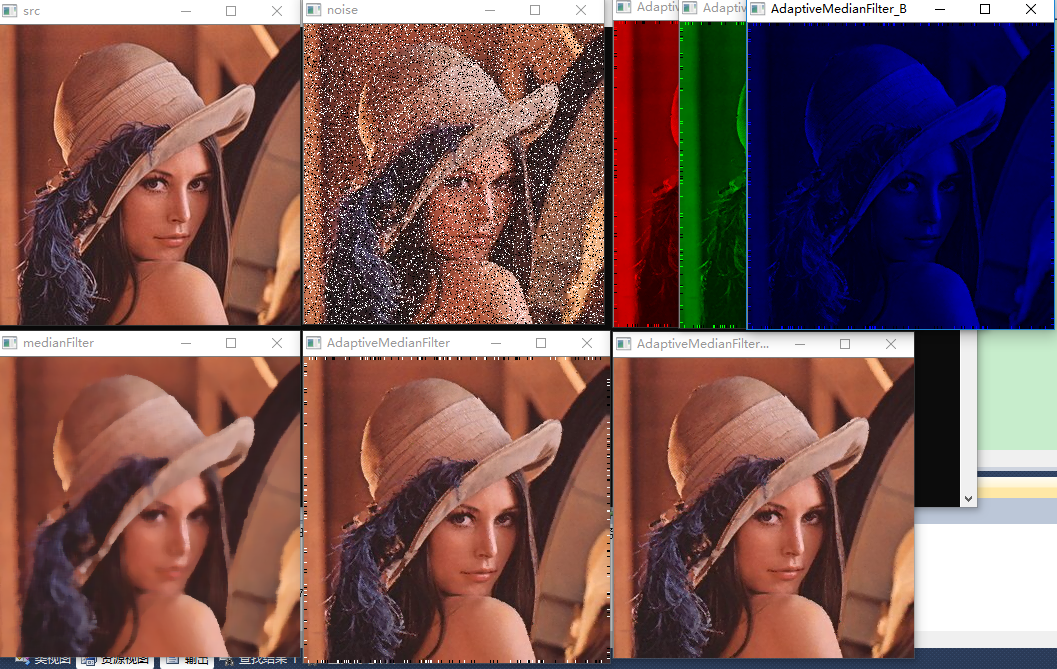上图中最后一张图是在自适应中值滤波处理后将扩展的边界去除后的效果。
自适应中值滤波原理
常规的中值滤波器的窗口尺寸是固定大小不变的，不能同时兼顾去噪和保护图像的细节。这时就要寻求一种改变，根据预先设定好的条件，在滤波的过程中，动态的改变滤波器的窗口尺寸大小，这就是自适应中值滤波器 Adaptive Median Filter。在滤波的过程中，自适应中值滤波器会根据预先设定好的条件，改变滤波窗口的尺寸大小，同时还会根据一定的条件判断当前像素是不是噪声，如果是则用邻域中值替换掉当前像素；不是，则不作改变。 自适应中值滤波器有三个目的： 滤除椒盐噪声 平滑其他非脉冲噪声 尽可能的保护图像中细节信息，避免图像边缘的细化或者粗化。
自适应中值滤波器不但能够滤除概率较大的椒盐噪声，而且能够更好的保护图像的细节，这是常规的中值滤波器做不到的。自适应的中值滤波器也需要一个矩形的窗口Sxy，和常规中值滤波器不同的是这个窗口的大小会在滤波处理的过程中进行改变（增大）。需要注意的是，滤波器的输出是一个像素值，该值用来替换点(x,y)处的像素值，点(x,y)是滤波窗口的中心位置。 在描述自适应中值滤波器时需要用到如下的符号： Zmin=Sxy中的最小灰度值 Zmax=Sxy中的最大灰度值 Zmed=Sxy中的灰度值的中值 Zxy表示坐标(x,y)处的灰度值 Smax=Sxy允许的最大窗口尺寸 自适应中值滤波器有两个处理过程，分别记为：A和B。 A : A1 = Zmed−Zmin A2 = Zmed−Zmax 如果A1 > 0 且 A2 < 0，跳转到 B； 否则，增大窗口的尺寸 （此处有两种情况：一种是 Zmed确实是噪点，此时模板太小无法分辨出噪点需要增大模板；另外一种是 Zmed是原图的有用点，但是A1 > 0 且 A2 < 0条件也不成立，需要增大模板，若直到最后窗口的尺寸≥Smax时条件任然不成立那么此时的Zmed则可以肯定是原图像中的点而不是噪点了） 如果增大后窗口的尺寸 ≤Smax，则重复A过程。否则，输出Zmed。 B: B1 = Zxy−Zmin B2 = Zxy−Zmax 如果B1 > 0 且 B2 < 0，则输出Zxy 否则输出Zmed（因为此时的Zmed不是噪点，Zxy 是噪点，用Zmed代替Zxy）。 自适应中值滤波原理说明： 过程A的目的是确定当前窗口内得到中值Zmed是否是噪声。如果Zmin<Zmed<Zmax，则中值Zmed不是噪声，这时转到过程B测试，当前窗口的中心位置的像素Zxy是否是一个噪声点。如果Zmin<Zxy<Zmax，则Zxy不是一个噪声，此时滤波器输出Zxy；如果不满足上述条件，则可判定Zxy是噪声，这是输出中值Zmed（在A中已经判断出Zmed不是噪声）。 从上面分析可知，当噪声出现的概率较低时，自适应中值滤波器使用较小的滤波核就可以得出结果，不需要去增加窗口的尺寸；反之，噪声的出现的概率较高，则需要增大滤波器的窗口尺寸，这些操作过程都是局部进行的，因此在对一幅图片滤波时，既有大滤波核滤波，也有小滤波核滤波，这就避免了中值滤波一刀切的问题。这也比较符合自适应中值滤波器的特点：噪声点比较多时，需要更大的滤波器窗口尺寸。
自适应中值滤波器代码实现
// Adaptive_Median_Filter.cpp : 定义控制台应用程序的入口点。
//

#include "stdafx.h"
#include "iostream"
#include "cv.h"
#include "highgui.h"

using namespace std;
using namespace cv;

//盐噪声
void saltNoise(Mat img, int n)
{
int x, y;
for (int i = 0;i < n / 2;i++)
{
x = rand() % img.cols;
y = rand() % img.rows;
if (img.type() == CV_8UC1)
{
img.at<uchar>(y, x) = 255;
}
else if (img.type() == CV_8UC3)
{
img.at<Vec3b>(y, x) = 255;
img.at<Vec3b>(y, x) = 255;
img.at<Vec3b>(y, x) = 255;
}
}
}

//椒噪声
void pepperNoise(Mat img, int n)
{
int x, y;
for (int i = 0;i < n / 2;i++)
{
x = rand() % img.cols;
y = rand() % img.rows;
if (img.type() == CV_8UC1)
{
img.at<uchar>(y, x) = 0;
}
else if (img.type() == CV_8UC3)
{
img.at<Vec3b>(y, x) = 0;
img.at<Vec3b>(y, x) = 0;
img.at<Vec3b>(y, x) = 0;
}
}
}

// 自适应中值滤波器
uchar adaptiveMedianFilter(Mat &img, int row, int col, int kernelSize, int maxSize)
{
vector<uchar> pixels;
for (int y = -kernelSize / 2;y <= kernelSize / 2;y++)
{
for (int x = -kernelSize / 2;x <= kernelSize / 2;x++)
{
pixels.push_back(img.at<uchar>(row + y, col + x));
}
}

sort(pixels.begin(), pixels.end());   //自动按从小到大顺序排序

auto min = pixels;
auto max = pixels[kernelSize*kernelSize - 1];
auto med = pixels[kernelSize*kernelSize / 2];
auto zxy = img.at<uchar>(row, col);
if (med > min && med < max)
{
// to B
if (zxy > min && zxy < max)
return zxy;
else
return med;
}
else
{
kernelSize += 2;
if (kernelSize <= maxSize)
return adaptiveMedianFilter(img, row, col, kernelSize, maxSize);// 增大窗口尺寸，继续A过程。
else
return med;
}
}

int main()
{
vector<Mat> RGBChannels(3);  //用于存储三通道
vector<Mat> RGBChannelsRemoveBorder(3);  //用于存储还原原始图片边界三通道

int MinSize = 3;
int MaxSize = 7;
int row=0;
int col=0;
Mat img;

imshow("src", img);
saltNoise(img, 20000);
pepperNoise(img,20000);
split(img,RGBChannels);  //将像素点的值分离为R、G、B 3个通道的值
imshow("noise", img);
Mat temp = img.clone();
//----------------自适应中值滤波------------
Mat img1,blank_ch,fin_img;
RGBChannelsRemoveBorder.create(RGBChannels.rows,RGBChannels.cols, CV_8UC1);  //存放滤波完成后去除扩充边界区域
RGBChannelsRemoveBorder.create(RGBChannels.rows,RGBChannels.cols, CV_8UC1);
RGBChannelsRemoveBorder.create(RGBChannels.rows,RGBChannels.cols, CV_8UC1);
vector<Mat> Channels_R(3),Channels_G(3),Channels_B(3),Channels_RGB(3);

for (int i=0;i<3;i++)   //分三个通道滤波
{
// 扩展图像的边界,自适应中值滤波内核最大为maxSize,边界扩充不能小于MaxSize/2
copyMakeBorder(RGBChannels[i], RGBChannels[i], MaxSize/2, MaxSize/2, MaxSize/2, MaxSize/2, BORDER_REPLICATE);
// 图像循环
for (int row = MaxSize / 2;row <  RGBChannels[i].rows - MaxSize / 2;row++)
{
for (int col = MaxSize / 2;col <  RGBChannels[i].cols - MaxSize / 2;col++)
{
RGBChannels[i].at<uchar>(row, col) = adaptiveMedianFilter(RGBChannels[i], row, col, MinSize, MaxSize);
}
}

// 图像边界还原
for (int row = 0;row <  RGBChannelsRemoveBorder[i].rows ;row++)
{
for (int col = 0;col <  RGBChannelsRemoveBorder[i].cols ;col++)
{
RGBChannelsRemoveBorder[i].at<uchar>(row, col) =RGBChannels[i].at<uchar>(row+MaxSize / 2, col+MaxSize / 2) ;
}
}

// 		blank_ch =Mat::zeros(Size(RGBChannels[i].cols, RGBChannels[i].rows), CV_8UC1);
// 		if (i==0)
// 		{
// 			Channels_B=RGBChannels[i];
// 			Channels_B=blank_ch;
// 			Channels_B=blank_ch;
// 			merge(Channels_B,fin_img);
// 		}
// 		else if(i==1)
// 		{
// 			Channels_G=blank_ch;
// 			Channels_G=RGBChannels[i];
// 			Channels_G=blank_ch;
// 			merge(Channels_G,fin_img);
// 		}
// 		else if(i==2)
// 		{
// 			Channels_R=blank_ch;
// 			Channels_R=blank_ch;
// 			Channels_R=RGBChannels[i];
// 			merge(Channels_R,fin_img);
// 		}
}
//-------------------------------------------
Channels_RGB=RGBChannels;
Channels_RGB=RGBChannels;
Channels_RGB=RGBChannels;
merge(Channels_RGB,fin_img);  //将像素点三通道值融合

Channels_RGB=RGBChannelsRemoveBorder;
Channels_RGB=RGBChannelsRemoveBorder;
Channels_RGB=RGBChannelsRemoveBorder;
merge(Channels_RGB,fin_img);  //将像素点三通道值融合

medianBlur(temp,temp,3);
imshow("medianFilter", temp);
waitKey();
destroyAllWindows();

return 0;
}


上述自适应中值滤波代码是对彩色图片进行滤波的操作方法，其实也就是将彩色图片的三通道分别进行滤波操作，滤波完成后再将三通道融合的过程。
展开全文OpenCV 图像滤波方法
• 针对单独的线性滤波和非线性滤波方法...实验表明, 该算法与标准自适应中值滤波算法和梯度倒数加权平滑算法相比, 能够很好地滤除高斯噪声、椒盐噪声, 以及两者的混合噪声, 并且对高强度的图像噪声具有明显的滤波优势。
• 像素点按一定的规律自适应地分组并赋予每组像素点相应的权重，最后采用加权中值滤波算法对检测出的噪声点进行滤波处理。 计算机模拟实验结果表明：该算法既能有效地滤除噪声，又能较好地保护图像细节，滤波性能比...椒盐去燥
• 自适应中值滤波算法有两个处理层次： **层次A：**若zmin<zmed<zmax，则转移到层次B； 否则，增Sxy尺 寸， 若Sxy<Smax，则重复层次A；否则，输出zmed。 **层次B：**若zmin<zxy<zmax，则输出zxy；否则...


文章目录
一、实现方法二、代码运行结果

一、实现方法
自适应中值滤波算法有两个处理层次： **层次A：**若zmin<zmed<zmax，则转移到层次B； 否则，增Sxy尺 寸， 若Sxy<Smax，则重复层次A；否则，输出zmed。 **层次B：**若zmin<zxy<zmax，则输出zxy；否则，输出zmed。 既然是中值滤波，那处理椒盐这类冲激噪声肯定比较合适，自适应中值滤波能在有效去除冲激噪声的基础上，平滑其他非冲激噪声，减少失真，保留图像细节，而对于同等大小的中值滤波器，则会损失较多的细节。原理性内容书中有详细的介绍~ 运行环境：Python+anaconda 直接上代码（因为是逐个像素操作，所以运行有点慢）

二、代码
# -*- coding:utf-8 -*-
"""
作者：YJH
日期：2021年10月19日
"""
import matplotlib.pyplot as plt
import cv2 as cv
import numpy as np
# from functools import reduce               # 导入reduce，将一个函数作用在一个序列上，并且序列内容自动累计

# 显示汉字
plt.rcParams['font.sans-serif'] = ['SimHei']
plt.rcParams['axes.unicode_minus'] = False

# 定义坐标数字字体及大小
def label_def():
plt.xticks(fontproperties='Times New Roman', size=8)
plt.yticks(fontproperties='Times New Roman', size=8)

# 读取图片
img_saltpep = cv.imread('ckt_saltpep_prob_pt25.tif', 0)               # 灰度图，噪声密度50%

def auto_median_filter(image, max_size):
origen = 3                                                        # 初始窗口大小
board = origen//3                                                 # 初始应扩充的边界
# max_board = max_size//2                                         # 最大可扩充的边界
copy = cv.copyMakeBorder(image, *[board]*4, borderType=cv.BORDER_DEFAULT)         # 扩充边界
out_img = np.zeros(image.shape)
for i in range(image.shape):
for j in range(image.shape):
def sub_func(src, size):                         # 两个层次的子函数
kernel = src[i:i+size, j:j+size]
# print(kernel)
z_med = np.median(kernel)
z_max = np.max(kernel)
z_min = np.min(kernel)
if z_min < z_med < z_max:                                 # 层次A
if z_min < image[i][j] < z_max:                       # 层次B
return image[i][j]
else:
return z_med
else:
next_size = cv.copyMakeBorder(src, **4, borderType=cv.BORDER_DEFAULT)   # 增尺寸
size = size+2                                        # 奇数的核找中值才准确
if size <= max_size:
return sub_func(next_size, size)     # 重复层次A
else:
return z_med
out_img[i][j] = sub_func(copy, origen)
return out_img

if __name__ == '__main__':                                       # 运行当前函数

img_auto_filter = auto_median_filter(img_saltpep, 7)
img_median = cv.medianBlur(img_saltpep, 7)
# img_re_median = cv.medianBlur(img_auto_filter, 3)                           # 两次3x3的中值滤波效果才能差不多
plt.subplot(131), plt.imshow(img_saltpep, "gray"), plt.title('椒盐噪声密度50%', fontsize='small'), label_def()
plt.subplot(132), plt.imshow(img_auto_filter, "gray"), plt.title('自适应中值滤波', fontsize='small'), label_def()
plt.subplot(133), plt.imshow(img_median, "gray"), plt.title('中值滤波', fontsize='small'), label_def()
# plt.subplot(144), plt.imshow(img_re_median, "gray"), plt.title('再中值滤波', fontsize='small'), label_def()

plt.show()

运行结果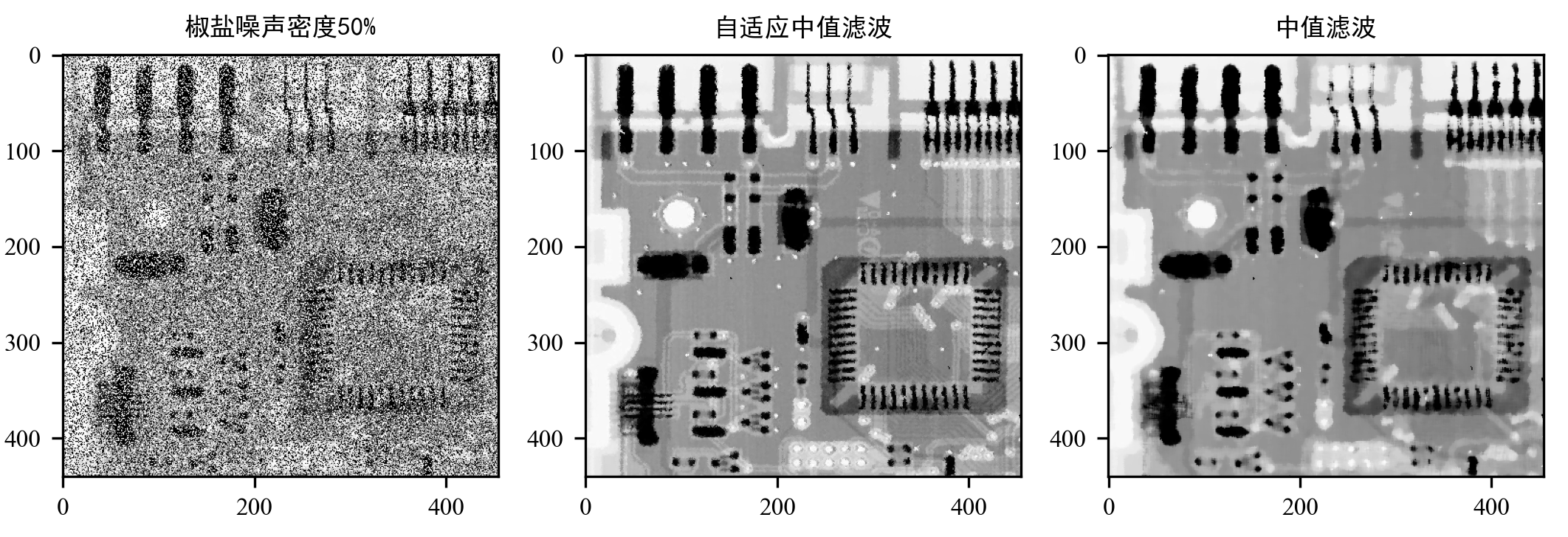效果还是挺好的 目前正在学习中，欢迎大家批评指正~
展开全文python opencv 图像处理
• 我自己写的图像处理以及中值滤波，可以处理BMP和JPG图片，包括灰度化，直方图，动态线性拉伸，图像翻转，插入文字，添加椒盐噪点，中值滤波，自适应中值滤波等各类功能，VC6中MFC写的。处理JPG图片需要自己去下GDI+...
• 中值滤波是一种使边缘模糊较轻的非线性滤波方法，是由Tukey发明的一种非线性信号处理技术，早期用于一维信号处理，后来很快被用到二维数字的图像平滑中。该算法不仅能够去除或减少随机噪声和脉冲噪声干扰，而且能够...
• 改下图片的路径，直接可以用，代码简单易懂，你值得拥有图像去噪
• 针对图像中存在的椒盐噪声，提出一种新的基于极值点检测的自适应中值滤波方法?该方法通过对极值点的检测确定图像中可能存在的噪声点，并标记噪声点；然后采用窗口尺度可伸缩的自适应中值滤波方法对噪声点进行滤波?......